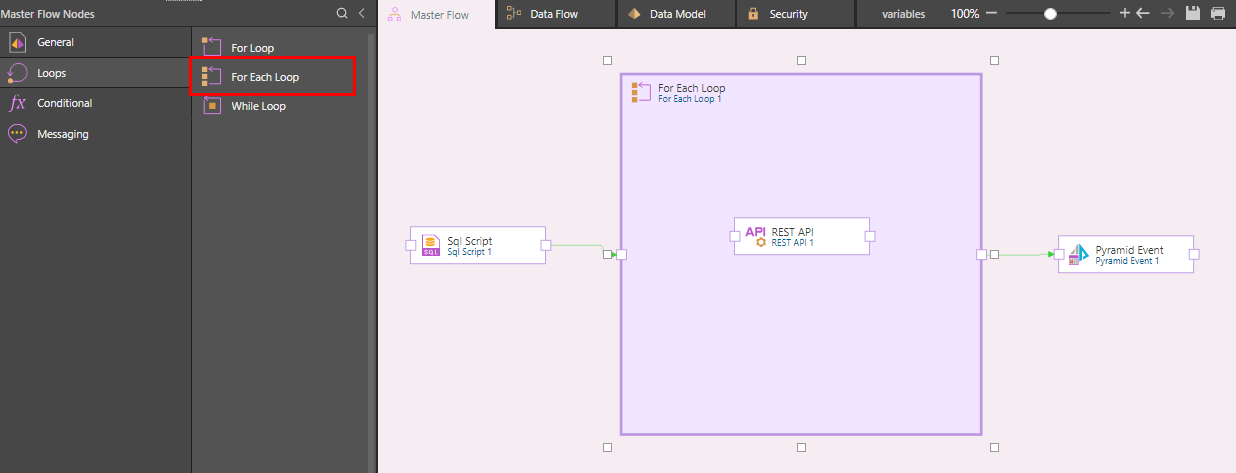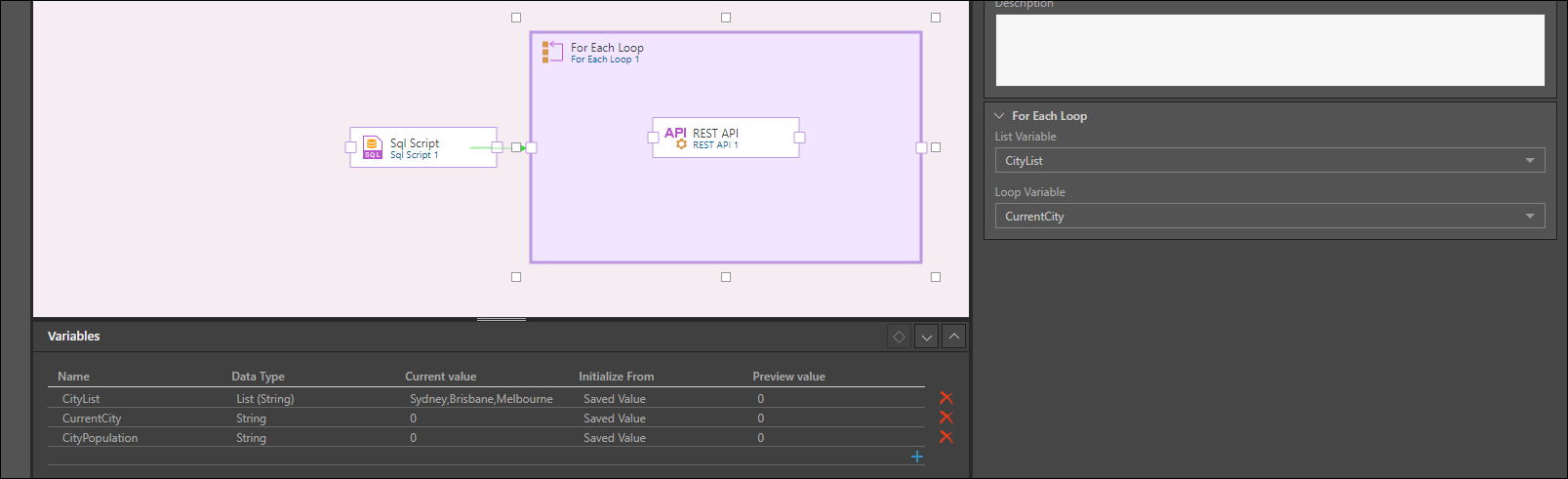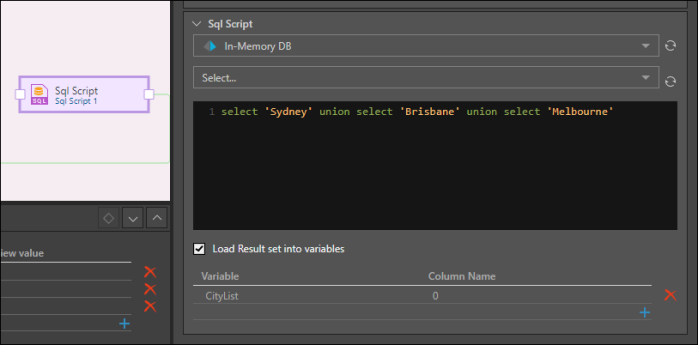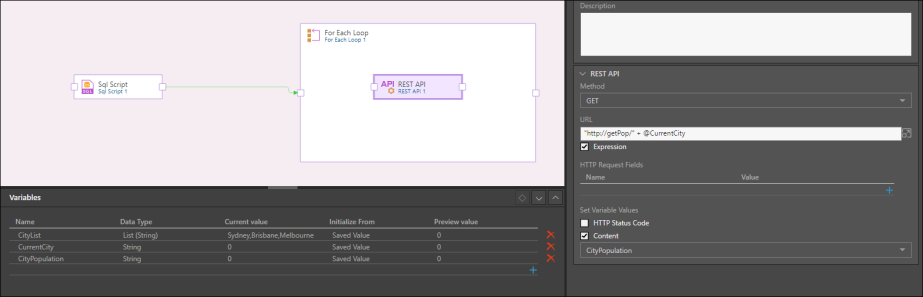# For Each Loop

The For Each loop is very similar to the For Loop, with the difference that there is no condition to be met. While the For Loop iterates through each row in the variable over and over again until the given condition resolves as false, the For Each Loop will run one time for each value in the list variable. Each time the loop runs, it iterates through the next row in the given list variable. Each value from the list is then loaded into the loop variable.## List Variables

Variables are used to store values that can then be passed into tables for incremental loading, and injected into scripts, filters, and calculated columns in the Data Flow. They can also be passed into containers, processes, and dynamic expressions in the Master Flow.

Variables can be used to store numeric values, text values, and date or date/ time values. They can be used to store a single value, or a list of values.

The For Each Loop is used to iterate through a list variable, which is a variable that stores a list of values. The For Each Loop will run as many times as there are list items in the variable; each time it runs it will load the current list value into the loop variable before running again. It will repeat this process until each list in the variable has been loaded into the loop variable.

• Click here to learn how to build a list variable from the Variables Panel.
• Click here to learn how to build a list variable from the SQL Script node in the Master Flow.

## For Each Loop Properties

The current value of the list variable is loaded into the loop variable before the loop is run.

To create a list variable, ensure that the List option is checked.

##### Display Name

The default display name of the for loop node is For Each Loop 1. Any subsequent for loop nodes are named according to this naming convention, with the appropriate numeric suffix, e.g. 'For Each Loop 2', 'For Each Loop 3', etc.

You can change the for loop node name from directly from this field.

##### Description

You have the option to add a description to each for each loop node; this can be a useful way of documenting the node for yourself and other users.

##### List Variable

Select the list variable that contains the list of values you want to load into the loop variable.

##### Loop Variable

Select the variable into which the list variable's values should be loaded. This cannot be a list variable.### Example

In the example below, an SQL script is used to load a set of 3 Australian cities into the list variable "CityList".The loop will run 3 times, each time loading the current list variable into the loop variable before running again.The REST API node is then used to get the population of the current city, which will then be loaded into the CityPopulation variable.